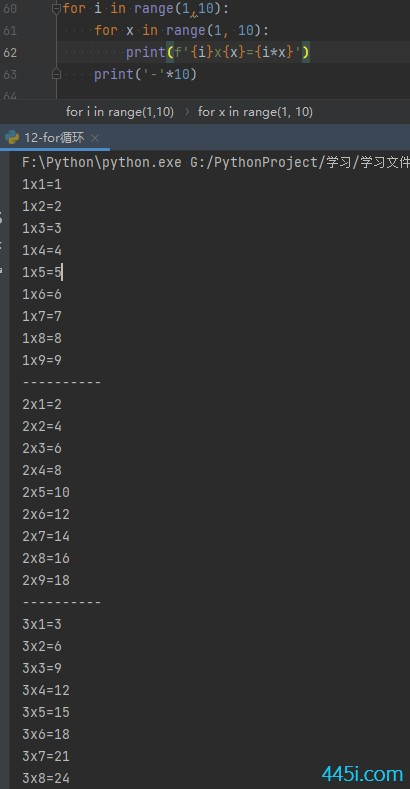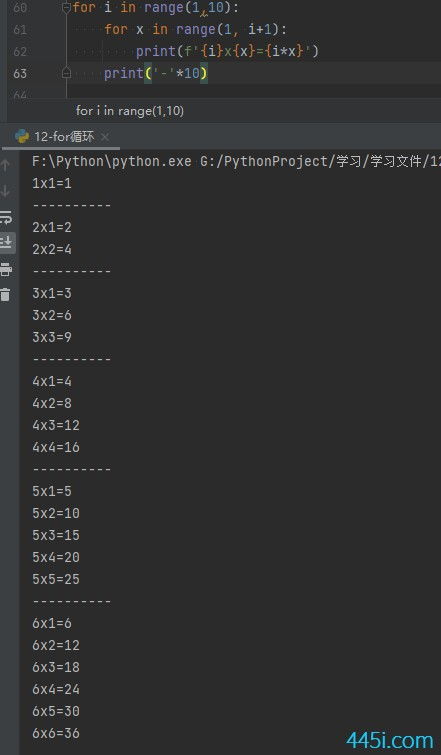# Python 用for循环写九九乘法表1

``````for i in range(1,10):
print('外层循环---->',i)
for x in range(1, 10):
print('内层循环-->', x)````````````for i in range(1,10):
for x in range(1, 10):
print(f'{i}*{x}={i*x}')
print('-'*10)````````````for i in range(1,10):
for x in range(1, i+1):
print(f'{i}x{x}={i*x}')
print('-'*10)``````### 觉得文章有用就打赏一下文章作者

#### 支付宝扫一扫打赏#### 微信扫一扫打赏